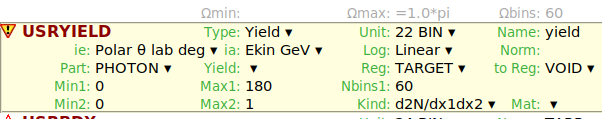# Angular distribution of USRYIELD

Dear Fluka Expert，

Now I want to measure the angular distribution of bremsstrahlung in the target，my settings and rusult are shown below.It is different from what I see in the courseware,It seems that I need to cosine the value of the x-axis.

I will upload my input file, please tell me what should I do.
tantalum_all.inp (1.8 KB)

Li

The double differential yield is obtained in"xx. lis" file, the unit here is GeV -1 Sr -1 per primary，If I want to get the x-axis as the angular interval and the y-axis as the yield (N), how should I calculate it?

Dear Fluka experts，

I used the USRYIELD card to get the double differential of the yield with respect to the angle （first quantity） and the energy（second quantity）, which unit is N Gev-1sr-1/pr , so I multiply the result by the solid angle bin width（in steradian） and energy bin width （although it is only one interval），then the result is the number of particles in each angular interval, is my understanding correct？

Li

Dear Li,

thank you for your question. With your USRYIELD card you are scoring a double differential yield of photons that leave the region TARGET and enter into the region VOID.

To have the normalized result, you should either provide the total crossing area (i.e. the area between your region TARGET and your region VOID) in the field Norm of the USRYIELD card or independently apply it during post-processing (see the Y Norm field in the plotting tab).

To obtain the “energy integrated” yield you would have to multiply by the energy bin width: since in your case it is 1 GeV, the result you get is already numerically equivalent to the “energy integrated” yield.

As specified in the manual, in case of polar angle quantities, the differential yield is always referred to the solid angle in steradian even if you specify it in degrees in the input file. So after proper normalization and multiplication by the energy bin width you have dN/dΩ in 1/cm^2/Sr/primary. When you do the plot, on the y axis you will have the yield dN/dΩ and on the x axis you will have by default the angle in radians that is subtending the corresponding solid angle, which should be what you wish to obtain.

If you want to obtain the number of particles in each angular interval, you have to multiply as well by the solid angle bin width.

I hope this was helpful. In case case of doubts do not hesitate to ask.
Best,
Davide

Dear Dacide ,

Thank you very much for your detailed explanation. This solves a lot of my doubts.
I have a question that is the surface area of the target should to be entered in the Norm item of the USRYIELD card? Or enter 1/area ? According to the unit of the latter result (cm-2), I think it is 1/area, but i’m not sure.
In a nutshell, after proper normalization, If i want to obtain the number of particles in each angular interval, I should multiply the result by the solid angle bin width (0.05235 In my practice) and then by the energy bin width (1GeV), and the unit of the final result is1/cm-2/primary . Is my understanding correct?
Thank you again.

Best,
Li

Dear Li,
in the Norm field you should enter the area, FLUKA will divide the result by that number. You can check that on top of the *_sum.lis file.

Mind that the output is a yield per solid angle (which is defined using the polar angles) so the bin width is not constant. From the *_tab.lis file you can use the first and second column which are the polar angles defining respectively the lower and upper values of the solid angles (with the relation 2π(1-cosθ)). The width of the solid angle bin will be2π(cosθ1 - cosθ2). If you are not convinced you can also calculate the cumulative yield and then check that what you get is consistent with what is written in the *_sum.lis file.

Best,
Davide

I fully understand now，thank you again.